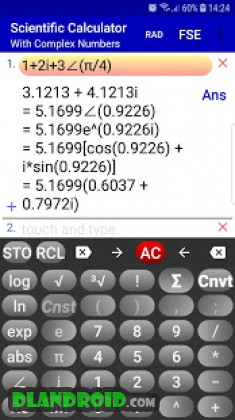Scientific Calculator | Complex Number Calculator 1.3.3 Apk Full Paid latest | Download Android

# Scientific Calculator | Complex Number Calculator 1.3.3 Apk Full Paid latest

event_note31 Aug 2019
android Apps, Tools

Scientific Calculator | Complex Number Calculator 1.3.3 Apk Full Paid latest is a Tools Android app

Download last version Scientific Calculator | Complex Number Calculator Apk Full Paid For Android with direct link

Scientific Calculator | Complex Number Calculator is a Tools android app made by gCalcD.com that you can install on your android devices an enjoy !

This scientific calculator handles complex numbers in a natural way. Any operation or function evaluation you can do with real numbers, you can also do the same with complex numbers by using this scientific complex number calculator. Namely, calculate expressions containing real, imaginary and, in general, any complex number in any form including, but not limited to, the standard (rectangular) and polar forms.

You can enter complex numbers in the standard (rectangular) or in the polar form. The calculated values will be displayed in standard form; optionally, this complex calculator displays the results in the polar form and other modular forms.

With a unique intuitive user interface this complex calculator computes arbitrary complicated complex number expressions with exceptional ease.

• Supports all standard mathematical functions with real, imaginary and complex numbers as their arguments including trigonometric, exponential, logarithmic, hyperbolic and inverse trig/hyperbolic functions plus the Gamma function, Γ, and Psi function, Ψ.

Swipe the number pad to the right to see more functions (trig, hyperbolic, inverses, etc.).

• Calculate expressions containing complex numbers in standard form a+bi and polar (phasor) form r∠(θ).

• Convert complex numbers from rectangular to polar and other modular forms and vice versa (long press i or ∠ to see all forms of the calculated result).

The complex number calculator allows the angle of polar form of complex numbers to be entered in radians or degrees.

• Fixed, scientific and engineering notations.

Do statistics with one or two dimensional real data:
• x1, x2 ,x3, … .
• Press Σ to calculate sum, mean, max, min, variance, sample and standard deviation, median, upper and lower quartile, etc.

• x1, y1; x2, y2; x3, y3; … .
• Press Σ to calculate the equation of the linear regression line and graph it.

• Long press ! to calculate combinations C(n, r) and permutations P(n, r).

• Enter functions f(x) or parametric equations p(t) and generate table of values.

• Physical/Chemical/Atomic and other scientific constants.

• Easy to use unit converter (Time, Mass, Length, Velocity, and many more).

Use this complex number calculator as a full scientific calculator to evaluate mathematical expressions containing real, imaginary and, in general, any complex numbers, and do more.

All of the above with more details are included in the built-in Instruction menu accompanying this scientific complex calculator.Scientific Calculator | Complex Number Calculator Apk Full

Whats New:

Crash fixes.
Performance improvement.

Rating and posting comments for this calculator on Google Play can help adding more features to this app.